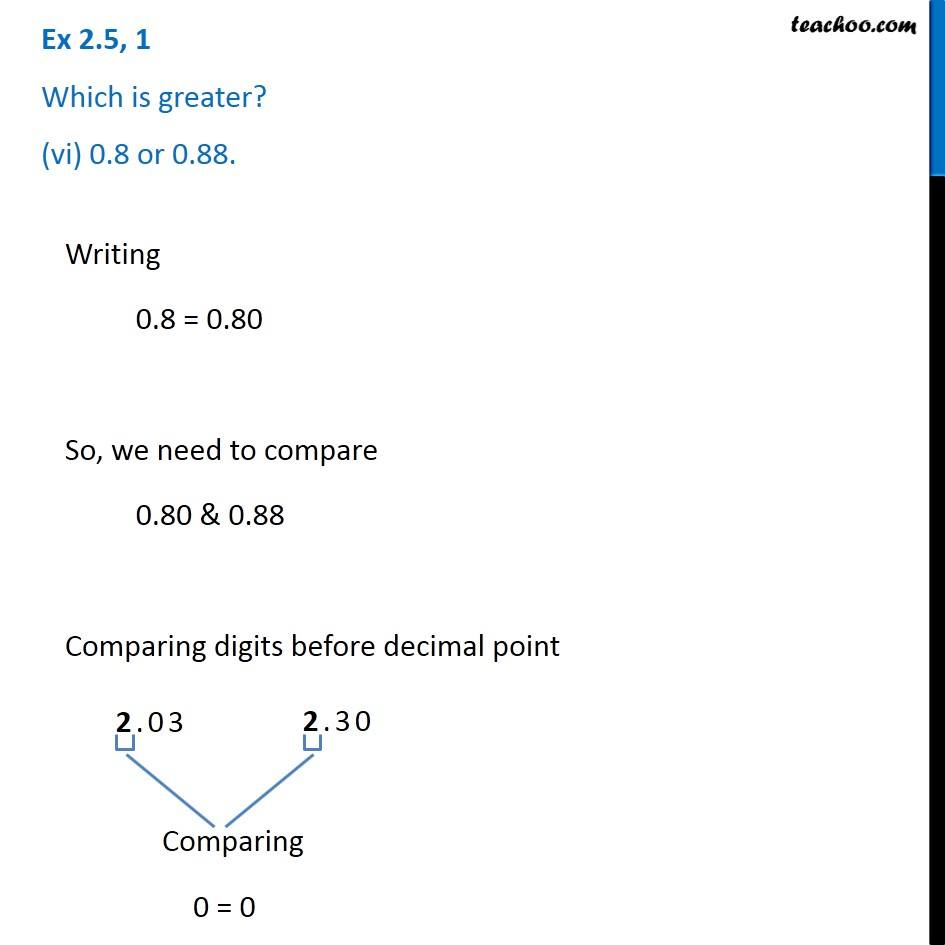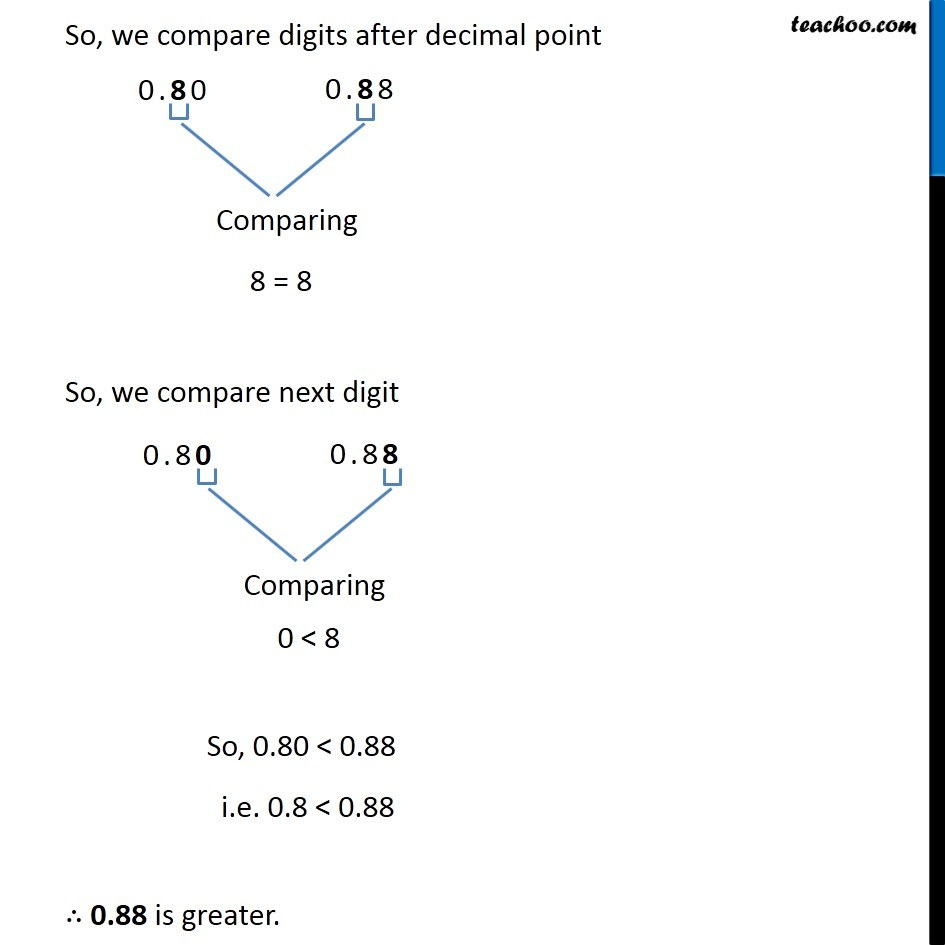1. Chapter 2 Class 7 Fractions and Decimals
2. Serial order wise
3. Ex 2.5

Transcript

Ex 2.5, 1 Which is greater? (vi) 0.8 or 0.88. Writing 0.8 = 0.80 So, we need to compare 0.80 & 0.88 Comparing digits before decimal point 0 = 0 So, we compare digits after decimal point 8 = 8 So, we compare next digit 0 < 8 So, 0.80 < 0.88 i.e. 0.8 < 0.88 ∴ 0.88 is greater.